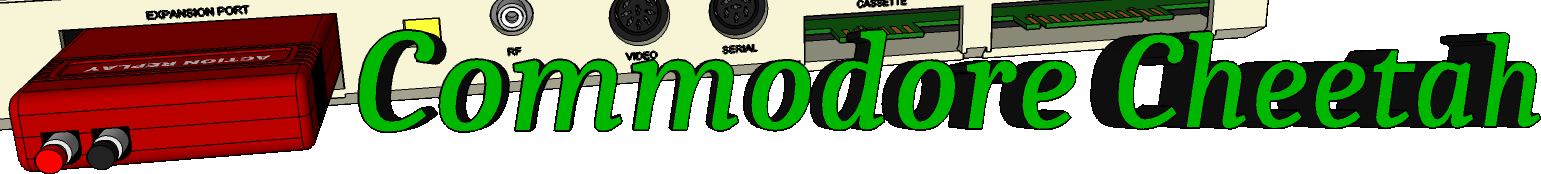Home PageeMail
email5-commodorecheetah.co.uk

Note: You will need to replace the '-' with the @ symbol.
This is to minimize spam.

 My BASIC Quick Reference Guide NOT Abbreviation: N TYPE: Logical Operator FORMAT: NOT Action: The NOT logical operator "complements" the value of each bit in its single operand, producing an integer "twos-complement" result. In other words, the NOT is really saying, "if it isn't. When working with a floating-point number, the operands are converted to integers and any fractions are lost. The NOT operator can also be used in a comparison to reverse the true/false value which was the result of a relationship test and therefore it will reverse the meaning of the comparison. In the first example below, if the "twos-complement" of "AA" is equal to "BB" and if "BB" is NOT equal to "CC" then the expression is true. EXAMPLES of NOT Operator: 10 IF NOT AA = BB AND NOT(BB = CC) THEN... NN% = NOT 96: PRINT NN% -97 NOTE: TO find the value of NOT use the expression X=(-(X+1)). (The two's complement of any integer is the bit complement plus one.

Commodore Cheetah made by Allen Monks, started in the year 2000.